All technical modulation and demodulation strive for greater power and/or efficiency of bandwidth in a white Gaussian additive stationary noise channel. Since bandwidth is a limited resource, one of the primary design goals of all the modulation schemes is to minimize the bandwidth required for transmission. On the other hand, spread spectrum techniques use a transmission bandwidth that is order of the magnitude greater than the bandwidth required the minimum signal.

The advantage of spread spectrum technique is that — many users can simultaneously use the same bandwidth without interfering with each other. Therefore, spread spectrum is not economic when the number of users is less.

• Spread spectrum is a form of wireless communications in which the frequency of the transmitted signal is deliberately varied resulting higher bandwidth.

• Spread-spectrum is apparent in the Shannon and Hartley channel-capacity theorem −

C = B × log2 (1 + S/N)

• In the given equation, `C’ is the channel capacity in bits per second (bps), which is the maximum data rate for a theoretical bit-error rate (BER). ‘B’ is the required channel bandwidth in Hz, and S/N is the signal-to-noise power ratio.

• Spread spectrum uses wideband, noise-like signals that are hard to detect, intercept, or demodulate. Additionally, spread-spectrum signals are harder to jam (interfere with) than narrow band signals.

• Since spread-spectrum signals are so wide, they transmit at a much lower spectral power density, measured in watts per hertz, than narrow band transmitters. Spread-spectrum and narrowband signals can occupy the same band, with little or no interference. This capability is the main attraction for all the interest in spread spectrum today.

Points to Remember

• The transmitted signal bandwidth is greater than the minimal information bandwidth, which is needed to transmit the signal successfully.

• Some function other than the information itself is normally employed to determine the resultant transmitted bandwidth.

Following are the two types of spread spectrum techniques −

• Direct Sequence and
• Frequency Hopping.

Direct Sequence is adopted by CDMA.

## Direct Sequence (DS)

Direct Sequence Code Division Multiple Access (DS-CDMA) is a technique to multiplex users by different codes. In this technique, the same bandwidth is used by different users. Each user is assigned with one its own spreading code. These sets of codes are divided into two classes −

• Orthogonal Codes and
• Non-Orthogonal Codes

Walsh sequences come into the first category which is Orthogonal Codes whereas other sequences i.e. PN, Gold, and Kasami are shift register sequences.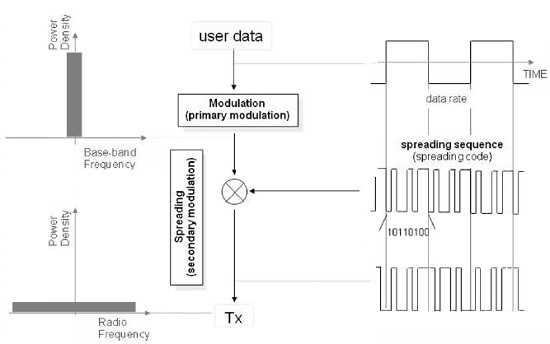Orthogonal codes are assigned to the users, the output of the correlator in the receiver will be zero except the desired sequence. In synchronous direct sequence, the receiver receives the same code sequence which was transmitted so that there is no time shift between the users.

### Demodulating DS Signals - 1

In order to demodulate DS signals, you need to know the code that was used at the time of transmission. In this example, by multiplying the code used in the transmission to the reception signal, we can get the transmitted signal.

In this example, multiple codes were used at the time of transmission (10,110,100) to the received signal. Here, we have calculated by using the law of two additives (Modulo 2 Addition). It is further demodulated by multiplying the code that was used at the time of this transmission, called the reverse diffusion (de-spreading). In the diagram given below, it can be seen that during the transmission of the data to the narrow band (Narrow Band) spectrum, the spectrum of the signal is dispread.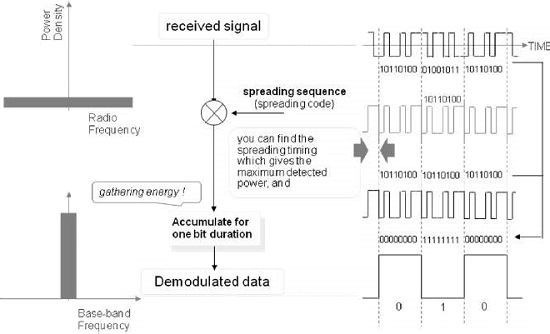### Demodulating DS Signals − 2

On the other hand, if you do not know the code that was used at the time of transmission, you will not be able to demodulate. Here, you are trying to demodulation in the code of different (10101010) and the time of transmission, but it has failed.

Even looking at the spectrum, it is spreading during the time of transmission. When it is passed through a band-pass filter (Band Path Filter), only this small signal remains and these are not demodulated.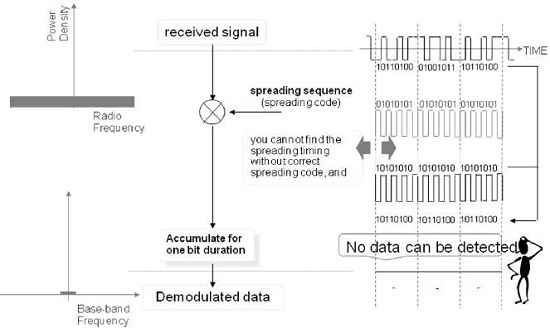As shown in the following figure, the power density of Spread Spectrum signals could be lower than the noise density. This is a wonderful feature that can keep the signals protected and maintain privacy.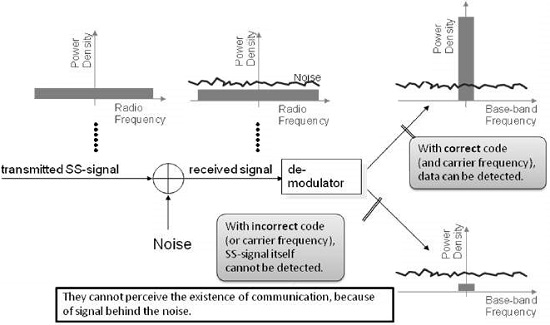By spreading the spectrum of the transmitted signal, one can reduce its power density such that it becomes less than the power density of the noise. In this way, it is possible to hide the signal in the noise. It can be demodulated if you know the code that was used to send the signal. In case the code is not known, then the received signal will remain hidden in the noise even after the demodulation.

### DS-CDMA

DS code is used in CDMA. So far, it has been explained basic part of the spread spectrum communication. From here, we will explain how Direct Sequence Code Division Multiple Access (DS-CDMA) works.

The signal which is spread spectrum, can be demodulated only by a code used for transmission. By using this, the transmission signal of each user can be identified by the separate code when it receives the signal. In the given example, the spread signal of the user A at the code A, and diffused signal of user B at code B. Each of the signal when it receives are mixed. However, by the inverse diffuser (Despreadder), it identifies the signal of each user.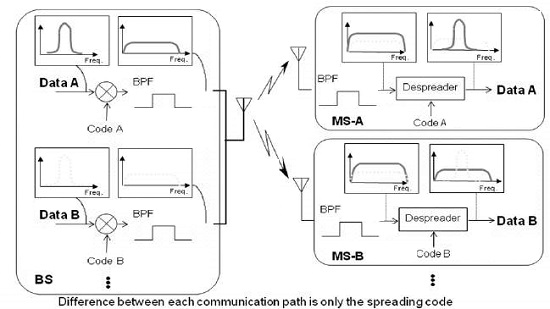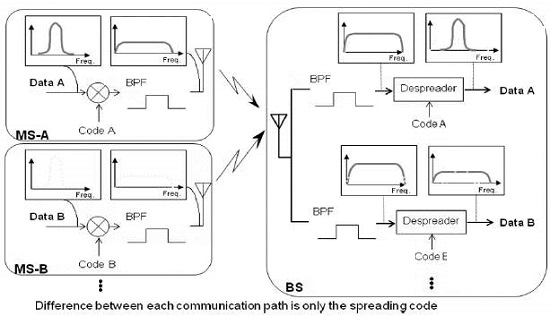Cross-Correlation

Correlation is a method of measurement of how precisely a given signal matches with a desired code. In CDMA technology, each user is assigned with a different code, the code which is being assigned or chosen by the user is very important to modulate the signal because it is related to the performance of the CDMA system.

One will get best performance when there will be clear separation between the signal of desired users and signals of the other users. This separation is made by correlating the desired signal code which was locally generated and other received signals. If the signal matches with the code of the user, then the correlation function will be high and the system can extract that signal. If the user's desired code has nothing in common with the signal, the correlation should be as close to zero as possible (thus eliminating the signal); also known as cross correlation. So, there is a self-correlation (Self-Correlation) and cross-correlation (Cross-Correlation).

Properties of self-correlation and code are shown in the diagram given below where correlation between spreading code ‘A’ and spreading code ‘B’ is shown. In this example, the calculated correlation of spreading code ‘A (1010110001101001) and spreading code ‘B’ (1010100111001001) is given, while performing calculations in below example, the result has come to 6/16.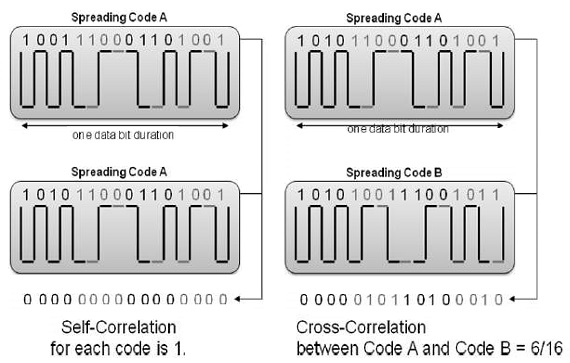Preferable Codes

Preferable code is used in CDMA. There are different codes that can be used depending on the type of a system of CDMA. There are two types of system −

• Synchronous (Synchronous) System and
• Asynchronous (Asynchronous) System.

In a synchronous system, orthogonal codes (Orthogonal Code) can be used. In asynchronous system for this, such as pseudo-random code (Pseudo-random Noise) or Gold code is used.

In order to minimize mutual interference in DS-CDMA, the spreading codes with less cross-correlation should be chosen.

Synchronous DS-CDMA

• Orthogonal Codes are appropriate. (Walsh code etc.)

Asynchronous DS-CDMA

• Pseudo-random Noise (PN) codes/Maximum sequence
• Gold Codes

Synchronous DS-CDMA

Synchronous CDMA Systems are realized in Point to Multi-point Systems. For example, Forward Link (Base Station to Mobile Station) in Mobile Phone.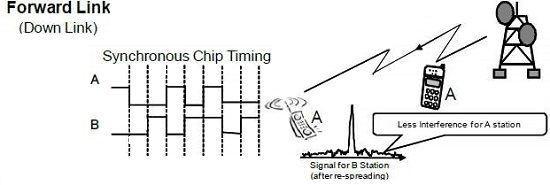Synchronization system is used in one-to-many (Point to Multipoint) systems. For example, at a given time, in a mobile communication system, a single base station (BTS) can communicate with multiple cell phones (forward link/downlink).

In this system, a transmission signal for all the users can communicate in synchronization. Means, "Synchronization" on this point is a sense that can be sent to align the top of each user signal. In this system, it is possible to use orthogonal codes and it is also possible to reduce mutual interference. And orthogonal codes, it is the sign, such as cross-correlation i.e. 0.

Asynchronous DS-CDMA

In asynchronous CDMA system, orthogonal codes have bad cross-correlation.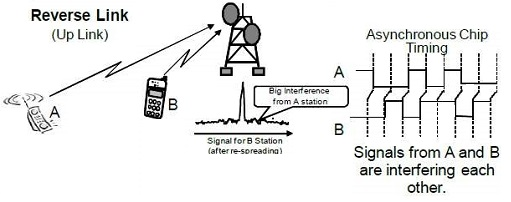Unlike the signal from the base station, the signal from the mobile station to the base station, becomes the asynchronous system.

In an asynchronous system, somewhat mutual interference increases, but it uses the other codes such as PN code or Gold code.

Since the signal is spread over a wide frequency band, the power spectral density becomes very low, so other communication systems do not suffer from this kind of communication. However, the Gaussian noise increases. Given below is a list of a few major advantages of Spread Spectrum −

• Multipath can be agreed with, as a large number of codes can be generated, allowing a large number of users.

• In spread spectrum, there is no limit of users whereas there is limitations of users in FDMA technology.

• Security − without knowing the spreading code, it is hardly possible to recover the transmitted data.

• Descending rejection − as large bandwidth is used the system; it is less susceptible to deformation.

## PN Sequence

The DS-CDMA system uses two types of spreading sequences, i.e., PN sequences and orthogonal codes. As mentioned above, the PN sequenc is generated by the pseudo-random noise generator. It is simply a binary linear feedback shift register, consisting of XOR gates and a shift register. This PN generator has the ability to create a sequence identical for both the transmitter and the receiver, and retaining the desirable properties of the noise randomness bit sequence.

A PN sequence has many features such as having an almost equal number of zeros and ones, very low correlation between shifted versions of the sequence, and very low cross-correlation with other signals such as interference and noise. However, it is able to correlate well with itself and its inverse. Another important aspect is the autocorrelation of the sequence as it determines the ability to synchronize and lock the spreading code for the received signal. This fight effectively effects the multiple interference and improves the SNR. M-sequences, Gold codes, and Kasami sequences are the examples of this class of sequences.

• A Pseudo-random Noise (PN) sequence is a sequence of binary numbers, e.g. ±1, which appears to be random; but it is in fact, perfectly deterministic.

• PN sequences are used for two types of PN spread spectrum techniques −

• Direct Signal Spread Spectrum (DS-SS) and

• Frequency Hop spread Spectrum (FH-SS).

• If ‘u’ uses PSK for modulating the PN sequence, it results in DS-SS.

• If ‘u’ uses FSK for modulating the PN sequence, it results in FH-SS.

## Frequency Hopping Technology

Frequency hopping is a spread spectrum in which the propagation takes place by hopping in frequency over a wide band. The precise order in which the break occurs is determined by a hopping table generated by using a pseudo-random code sequence.

Hopping rate is a function of the speed information. The order of frequencies is selected by the receiver and is dictated by the pseudo-random noise sequence. Although the transmission of a frequency hopping signal spectrum is quite different from that of a direct sequence signal, it suffices to note that the data is distributed over a signal band is larger than necessary to carry. In both the cases, the resulting signal will appear as noise and the receiver uses a similar technique, which is used in the transmission to recover the original signal.